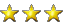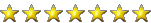Drawing fractals patterns with JavaScript canvas

PooPipeBoyThis is an interesting programming project I did a few years ago when I was super interested in playing with code.
The "coolFractals" program was originally not mine (it was an example program included with Just Basic) but my idea was to port it to JavaScript.
Obviously the benefits of HTML is that the code can now be run on any computer using just a browser.
Anyone who's interested in experimenting with it, just copy the code into Notepad and save it with a ".html" extension.

'coolFractal.bas contributed by Rod
nomainwin
dim col\$(12)
WindowWidth = DisplayWidth
WindowHeight = DisplayHeight
UpperLeftX = int((DisplayWidth-WindowWidth)/2)
UpperLeftY = int((DisplayHeight-WindowHeight)/2)
midx=int(WindowWidth/2)
midy=int(WindowHeight/2)
button #1, "Clear", [clear], LR, 50, 10
open "Fractals" for graphics_nsb as #1
print #1, "trapclose [quit]"

[clear]
print #1, "down ; fill black"

[draw]
'set up some random colors
for c=0 to 12
col\$(c)=str\$(int(rnd(0)*256))+" "+str\$(int(rnd(0)*256))+" "+str\$(int(rnd(0)*256))
next c

'set up some random starting positions
a=rnd(0)
b=0.9998
c=2-2*a
dots=12000
x=j=0
y=rnd(0)*12+0.1

'calculate and draw the points
for i=0 to dots
scan
z=x
x=b*y+j
j=a*x+c*(x^2)/(1+x^2)
y=j-z
xp=(x*20)+midx
yp=(y*20)+midy
print #1, "color ";col\$(i/1000)
print #1, "set ";xp;" ";yp
next i
goto [draw]

[quit]
close #1
end

My updated version of the code for HTML:

HTML:
<!DOCTYPE html>
<html>

<body>

<canvas id="myCanvas" style="border:1px solid #000000">
</canvas>

<script language="javaScript">

//Variables
var a;
var b;
var c;
var x;
var y;
var z;
var j;
var dots;
var xp;
var yp;
var i;
var colors;
var randomColour;
var canvas = document.getElementById("myCanvas");
var context = canvas.getContext("2d");

//Resize and fill in canvas
canvas.width = screen.width;
canvas.height = screen.height;
context.fillStyle = "#000000";
context.fillRect(0, 0, canvas.width, canvas.height);

//Run code immediately to remove timeout() delay.
//Set up some random starting positions
a = Math.random();
b = 0.9998;
c = 2 - 2*a;
dots = 80000;
x = 0;
j = 0;
y = Math.random()*12 + 0.1;
//Calculate and draw the points
i = 0;
function draw() {
if (i<=dots) {
z = x;
x = b*y+j;
j = a*x+(c*(Math.pow(x,2)))/(1+Math.pow(x,2));
y = j-z;
xp = (x*20) + (canvas.width/2);
yp = (y*20) + (canvas.height/2);
context.fillRect(xp, yp, 2, 2);
i++;
setTimeout(draw);
};
};
setTimeout(draw)
timeout();

function timeout() {
//Generate a random hex colour
colors = ["#FF0000","#FFD700","#008000","#0000FF"]
randomColour = colors[Math.floor(Math.random() * colors.length)];
context.fillStyle = randomColour;
setTimeout(function () {
//Set up some random starting positions
a = Math.random();
b = 0.9998;
c = 2 - 2*a;
dots = 50000;
x = 0;
j = 0;
y = Math.random()*12 + 0.1;
//Calculate and draw the points
i = 0;
function draw() {
if (i<=dots) {
z = x;
x = b*y+j;
j = a*x+(c*(Math.pow(x,2)))/(1+Math.pow(x,2));
y = j-z;
xp = (x*20) + (canvas.width/2);
yp = (y*20) + (canvas.height/2);
context.fillRect(xp, yp, 2, 2);
i++;
setTimeout(draw);
};
};
setTimeout(draw)
timeout();
}, 6000);
}

timeout();

</script>

</body>
</html>
Screenshot:Last edited:
•biffzinker

Solaris17

Dainty Moderator
Staff member
This is cool thanks for sharing.

•biffzinker and PooPipeBoy

PooPipeBoyThis is cool thanks for sharing.
No worries. I wouldn't mind making it run 2x faster, but otherwise it's working pretty good.

biffzinker•Solaris17

Staff member

PooPipeBoyPegs the RTX 2060 more than the Ryzen 3600
Man that's crazy, I never noticed the GPU usage before. I guess the canvas operations become quite graphics-intensive when it's constantly being updated.